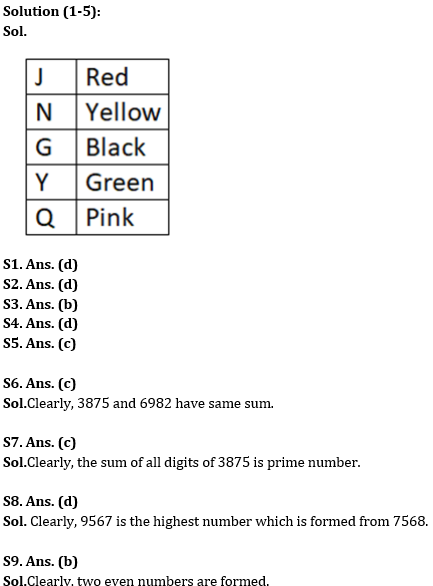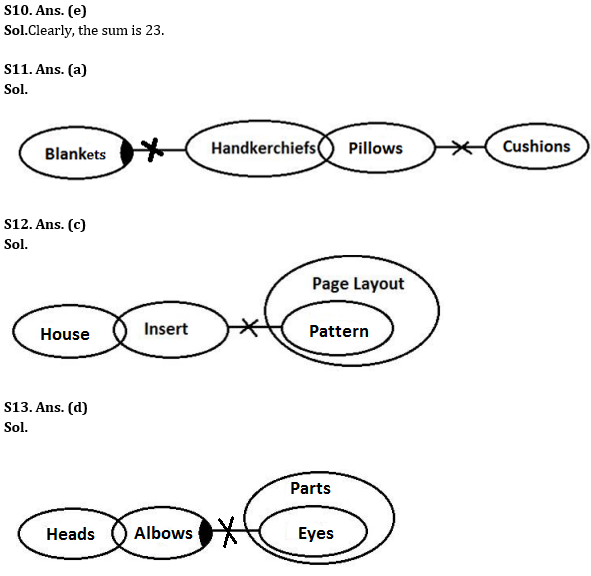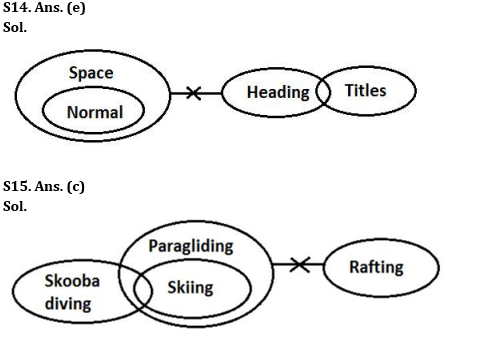Latest Banking jobs   »

# Reasoning Ability Quiz For RBI Grade B/ ECGC PO/ SIDBI Grade A Prelims 2022- 16th May

Directions (1-5): Study the following information carefully and answer the questions accordingly.
Five boxes of different colors are placed one above the other in a stack. Y is not Black. J is placed immediately above N but is not Yellow. One box is placed between G and the Pink box. One box is placed between Y and the Yellow box. Q is not green. One box is placed between G and the Red box. Two boxes are placed between Yellow and the Pink box.

Q1. Which of the following boxes is green color?
(a) The box placed above N
(b) G
(c) The box placed at the bottom
(d) Y
(e) None of these

Q2. How many boxes are placed below N?
(a) Four
(b) Two
(c) One
(d) Three
(e) None

Q3. What is the color of the box placed between Y and N?
(a) Yellow
(b) Black
(c) Green
(d) Pink
(e) None of these

Q4. Which of the following boxes is placed at the top?
(a) Yellow box
(b) Y
(c) Q
(d) Red box
(e) None of these

Q5. Which of the given combination is correct?
(a) N – Red
(b) Q – Yellow
(c) G – Black
(d) J – Green
(e) Y – Pink

Directions (6-10): The following questions are based on the five four-digit numbers given below.

6982   3875   3267   4932   7568

Q6. If the first and fourth digits of each of the numbers are added, then the resulting sum of which of the following numbers will be the same?
(a) 4932, 3267
(b) 3267, 3875
(c) 3875, 6982
(d) 7568, 6982
(e) 6982, 7568

Q7. If all the digits of each of the numbers are added, then the resulting sum of which of the following numbers will be a prime number?
(a) 4932
(b) 3267
(c) 3875
(d) 7568
(e) 6982

Q8. If one is added to the last digit of each number and then the positions of the first and the fourth digits are interchanged, then which of the following will be the highest number?
(a) 4932
(b) 3267
(c) 3875
(d) 7568
(e) 6982

Q9. If in each number second and fourth digits are interchanged, then how many even numbers are formed?
(a) One
(b) Two
(c) Three
(d) Four
(e) None

Q10. If four digits arranged in descending order within the number then, what is sum of digits of second lowest number?
(a) 28
(b) 29
(c) 21
(d) 24
(e) 23

Directions (11-15): In the question below three statements are given followed by the conclusions. You have to take the given statements to be true even if they seem to be at variance with commonly known facts. Read all the conclusions and then decide which of the given conclusions logically follows from the given statements disregarding commonly known facts.

Q11. Statements: Some Blankets are not Handkerchiefs. Only a few Handkerchiefs are Pillows. No Pillows are Cushions.
Conclusions:
I. Some Handkerchiefs are not Cushions.
II. Some Blankets are not Pillows
(a) Only I follows
(b) Only II follows
(c) Either I or II follows
(d) Neither I nor II follows
(e) Both I and II follow

Q12. Statements: Only a few House are Insert. No Insert are Pattern. All Patterns are Page Layouts.
Conclusions:
I. Some House are Page Layout.
II. No House are Page Layout.
(a) Only I follows
(b) Only II follows
(c) Either I or II follows
(d) Neither I nor II follows
(e) Both I and II follow

Q13. Statements: Only a few Heads are Albows. Some Albows are not Eyes. All Eyes are Parts.
Conclusions:
I. Some Heads are not Eyes.
II. Some Parts are not Albows.
(a) Only I follows
(b) Only II follows
(c) Either I or II follows
(d) Neither I nor II follows
(e) Both I and II follow

Q14. Statements: All Normal are Space. No Space are Heading. Only a few Headings are Titles.
Conclusions:
II. Some Titles are not Space
(a) Only I follows
(b) Only II follows
(c) Either I or II follows
(d) Neither I nor II follows
(e) Both I and II follow

Q15. Statements: Only a few Skooba diving are Skiing. All Skiing are Paragliding. No Paragliding is Rafting.
Conclusions:
I. Some Rafting are Skooba diving.
II. No Rafting are Skooba diving.
(a) Only I follows
(b) Only II follows
(c) Either I or II follows
(d) Neither I nor II follows
(e) Both I and II follow

Solutions#### Congratulations!